# Probability of intersection

Three students have a probability of 0.7,0.5 and 0.4 to graduated from university respectively. What is the probability that at least one of them will be graduated?

Correct result:

p =  0.91

#### Solution: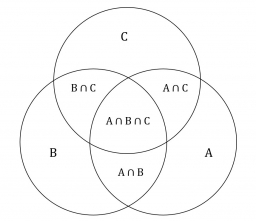We would be pleased if you find an error in the word problem, spelling mistakes, or inaccuracies and send it to us. Thank you!Tips to related online calculators
Would you like to compute count of combinations?

## Next similar math problems:

• Three students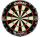Three students independently try to solve the problem. The first student will solve a similar problem with a probability of 0.6, the second student will solve at a probability of 0.55, and the third will solve at a probability 0.04. The problem is resolve
• The accompanyingThe accompanying table gives the probability distribution of the number of courses randomly selected student has registered Number of courses 1 2 3 4 5 6 7 Probability 0.02 0.03 0.1 0.3 0.4 - 0.01 respectively. a) Find the probability of a student registe
• Three shooters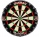Three shooters shoot, each one time, on the same target. The first hit the target with a probability of 0.7; second with a probability of 0.8 and a third with a probability of 0.9. What is the probability to hit the target: a) just once b) at least once c
• DigitsHow many five-digit numbers can be written from numbers 0.3,4, 5, 7 that is divided by 10 and if digits can be repeated.
• Double probabilityThe probability of success of the planned action is 60%. What is the probability that success will be achieved at least once if this action is repeated twice?
• Lottery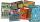Fernando has two lottery tickets each from other lottery. In the first is 973 000 lottery tickets from them wins 687 000, the second has 1425 000 lottery tickets from them wins 1425 000 tickets. What is the probability that at least one Fernando's ticket
• Phone numbersHow many 7-digit telephone numbers can be compiled from the digits 0,1,2,..,8,9 that no digit is repeated?
• Dices throwsWhat is the probability that the two throws of the dice: a) Six falls even once b) Six will fall at least once
• Lion or virgin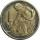We toss coin, every throw fits lion or a virgin with equal probability 1/2. Determine how much at least we have to make throws that with probability 0.9 lion fell at least once.
• Chambers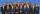The decision-making committee consists of three people. In order for the commission's decision to be valid, at least two members must vote in the same way. It is not possible not to vote in the commission, everyone only votes yes or no. We assume that the
• The universityAt a certain university, 25% of students are in the business faculty. Of the students in the business faculty, 66% are males. However, only 52% of all students at the university are male. a. What is the probability that a student selected at random in the
• Study results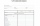There are 7 students in the class with excellent results, 6 praiseworthy, 5 good, 4 sufficient and 3 insufficient students. What is the probability that it will be a good student when summoned?
• Set of coordinatesConsider the following ordered pairs that represent a relation. {(–4, –7), (0, 6), (5, –3), (5, 2)} What can be concluded of the domain and range for this relation?
• FamilyWhat is the probability that a family with 7 childrens have: exactly 5 girls? 7 girls and 0 boys? Consider the birth probability of a girl is 48.69% and boy 51.31%.
• Air draftThe numbers 1,2,3,4,5 are written on five tickets on the table. Air draft randomly shuffled the tickets and composed a 5-digit number from them. What is the probability that he passed: and, the largest possible number b, the smallest possible number c, a
• Three-digit numbersWe have digits 0,1,4,7 that cannot be repeated. How many three-digit numbers can we write from them? You can help by listing all the numbers.
• Normal DistributionAt one college, GPA's are normally distributed with a mean of 3.1 and a standard deviation of 0.4. What percentage of students at the college have a GPA between 2.7 and 3.5?